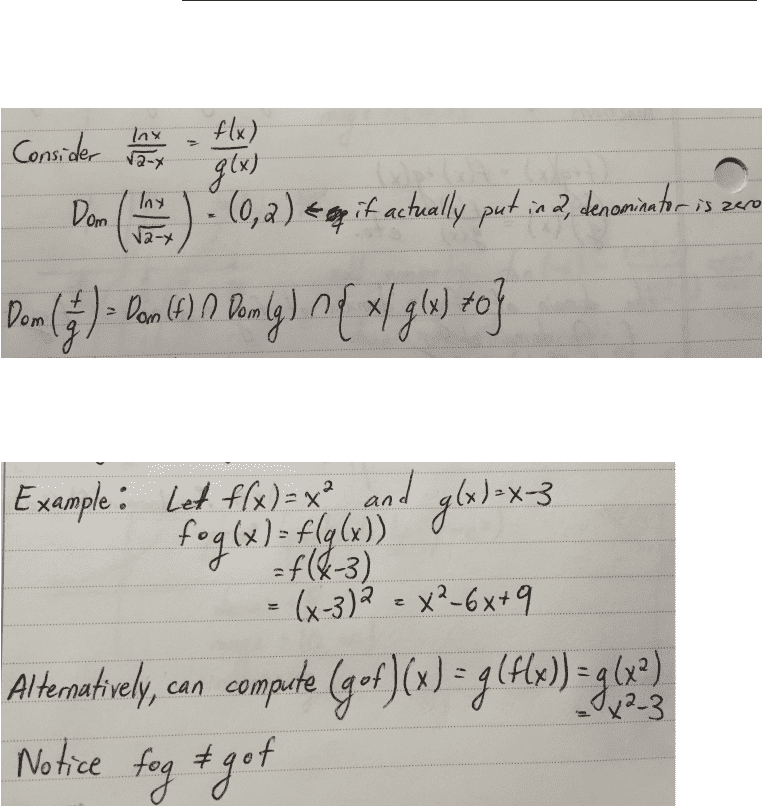Class Notes (1,100,000)
CA (630,000)
UTSG (50,000)
MAT (4,000)
MAT135H1 (700)
Lecture 3

# MAT135H1 Lecture Notes - Lecture 3: Function Composition, Inverse Function

Department
Mathematics
Course Code
MAT135H1
Professor
Alison Smith
Lecture
3

This preview shows page 1. to view the full 4 pages of the document.MAT135 - Lecture 3 - Composite and Inverse Functions
New functions from old functions (cont’d from lecture 2)
Remember: domain of (f + g) = domain of (f - g) = domain of (f · g) = domain of f AND domain
of g (i.e. overlapping area)
Composite Functions
Given two functions f and g, the composite function f g is given by (f g)(x) = f(g(x))
Domain of composite functions